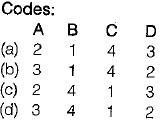Courses

# Basic Concept - 2

## 10 Questions MCQ Test Topicwise Question Bank for GATE Mechanical Engineering | Basic Concept - 2

Description
This mock test of Basic Concept - 2 for Mechanical Engineering helps you for every Mechanical Engineering entrance exam. This contains 10 Multiple Choice Questions for Mechanical Engineering Basic Concept - 2 (mcq) to study with solutions a complete question bank. The solved questions answers in this Basic Concept - 2 quiz give you a good mix of easy questions and tough questions. Mechanical Engineering students definitely take this Basic Concept - 2 exercise for a better result in the exam. You can find other Basic Concept - 2 extra questions, long questions & short questions for Mechanical Engineering on EduRev as well by searching above.
QUESTION: 1

Solution:
QUESTION: 2

Solution:
QUESTION: 3

### Match List-I with List-I I and select the correct answer: List-I A. Interchange of matter is not possible in a B. Any processes in which the system returns to its original condition or state is called C. Interchange of matter is possible in a D. The quantity of matter under consideration in thermodynamics is called List-ll 1. Open system 2. System 3. Closed system 4. CycleSolution:
QUESTION: 4

Which one of the following represents open thermodynamic system?

Solution:
QUESTION: 5

A thermodynamic system is considered to be an isolated one if

Solution:
QUESTION: 6

A control volume is

Solution:
QUESTION: 7

Athermodynamic system refers to

Solution:

A certain quantity of matter or a region in space  upon which attention is focused in the analysis of a problem is called a system.

QUESTION: 8

In highly rarefied gases, the concept of this loses validity

Solution:

The concept of continuum loses validity when the mean free path of the molecules approaches the order of magnitude of the dimension of the vessel. So, in highly rarefied gases the concept of continuum loses its validity.

QUESTION: 9

Which of the following is an example of heterogeneous system?

Solution:
QUESTION: 10

Consider the following:
1. Temperature
2. Viscosity
3. Specific entropy
4. Thermal conductivity

Which of the above properties of a system is/are intensive

Solution: